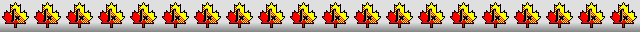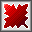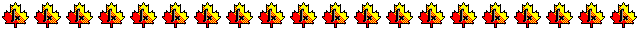Maple worksheets on the derivation of order 9 Runge-Kutta schemesDerivation of 16 stage, combined order 8 and 9 Runge-Kutta schemes - RKcoeff9a.mws

• Schems by Verner, Sharp and modifications of two schemes by Tsitouras.
See:
"Explicit Runge-Kutta methods with estimates of the Local Truncation Error", by J.H. Verner,
SIAM Journal of Numerical Analysis 1978, 772-790,
"High order explicit Runge-Kutta pairs for ephemerides of the Solar System and the Moon", by Philip W. Sharp,
Journal of Applied Mathematics & Decision Sciences, 4(2), 183-192 (2000),
"Optimized explicit Runge-Kutta pair of order 9(8)", by Ch. Tsitouras,
Applied Numerical Mathematics, 38 (2001)  123-134.

• Coefficients for the schemes and some properties
RKcoeff9a_1.pdf     RKcoeff9a_2.pdf     RKcoeff9a_3.pdf     RKcoeff9a_4.pdf     RKcoeff9a_5.pdf

Derivation of 16 stage, combined order 8 and 9 Runge-Kutta schemes - RKcoeff9b.mws

• Two schemes of Tsitouras
See: "Optimized explicit Runge-Kutta pair of order 9(8)", by Ch. Tsitouras,
Applied Numerical Mathematics, 38 (2001)  123-134.

• Coefficients for the schemes and some properties
RKcoeff9b_1.pdf     RKcoeff9b_2.pdf

Derivation of 17 stage, combined order 8 and 9 Runge-Kutta schemes - RKcoeff9c.mws     RKcoeff9d.mws

Procedures related to the construction of Runge-Kutta schemes - butcher.zip

Procedures for root-finding and determining minimum points  - roots.zip

Procedures for numerical integration  - intg.zip

Top of page

Main index